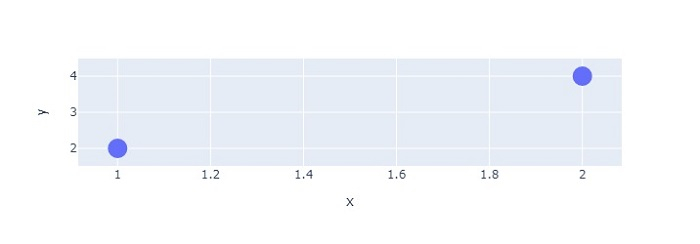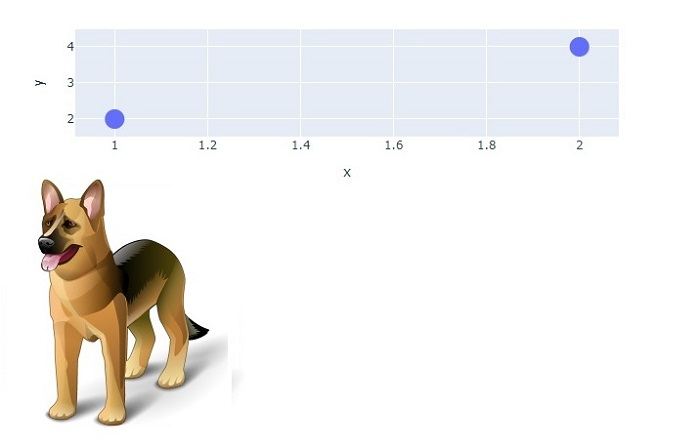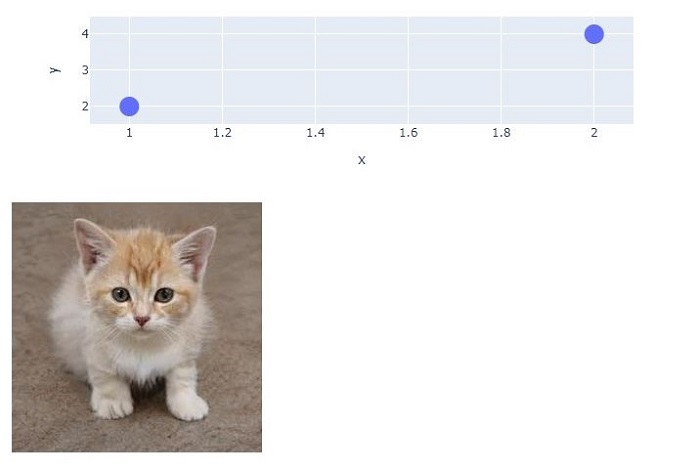# How to display an image on hovering over a point in Python Plotly?

Plotly is an open-source plotting library in Python that can generate several different types of charts. Python users can use Plotly to create interactive web-based visualizations that can be displayed in Jupyter notebooks, saved to standalone HTML files, or served as a part of web applications using Dash. Plotly can also be used in static document publishing and desktop editors such as PyCharm and Spyder.

Dash is a Python framework and it is used to create interactive web-based dashboard applications. The dash library adds all the required libraries to web-based dashboard applications.

In this tutorial, we will show how you can add multiple graphs to a Plotly Dash app on a single browser page.

Follow the steps given below to generate Dash app on a single page.

Step 1

Import the Dash library.

import dash


Step 2

Import the Dash core components, dcc and html. We will use the dcc.Graph() method to set the style for height and width coordinates.

from dash import dcc, html


Step 3

Import the dash dependencies using the below module.

from dash.dependencies import Input, Output


Step 4

Import the plotly.express module and alias as px. We will use this method to generate graphs.

import plotly.express as px


Step 5

Generate a dataset using the Pandas module.

#generate dataframe
df = pd.DataFrame(
dict(
x=[1, 2],
y=[2, 4],
images=[dogImage,catImage],
)
)


Step 6

Set the images from specific URLs. The sample URL is defined below −

dogImage = "data:image/png;base64,
catImage = "data:image/png;base64,


Step 7

Create a scatter plot with the X and Y coordinates −

# create scatter plot with x and y coordinates
fig = px.scatter(df, x="x", y="y",custom_data=["images"])


Step 8

Create the main function to run the App server using the following command −

app = dash.Dash(__name__)
if __name__ == '__main__':
app.run_server(debug=True)


Step 9

Use the update_layout() method to perform click mode and set the update_traces() method to perform marker size.

# Update layout and update traces
fig.update_layout(clickmode='event+select')
fig.update_traces(marker_size=20)


Step 10

Generate the App layout to show the Dash graph. It is defined below,

# Create app layout to show dash graph
app.layout = html.Div(
[
dcc.Graph(
id="graph_interaction",
figure=fig,
),
html.Img(id='image', src='')
]
)


Step 11

Create the callback() function to hover the data on specific coordinates, as shown below −

@app.callback(
Output('image', 'src'),
Input('graph_interaction', 'hoverData'))

def open_url(hoverData):
if hoverData:
return hoverData["points"]["customdata"]
else:
raise PreventUpdate

# Create app layout to show dash graph
app.layout = html.Div(
[
dcc.Graph(
id="graph_interaction",
figure=fig,
),
html.Img(id='image', src='')
]
)


## Example

The complete code to display an image on hovering dash graph,

import dash
from dash.exceptions import PreventUpdate
from dash import dcc, html
from dash.dependencies import Input, Output
import plotly.express as px
import pandas as pd

# Create dash app
app = dash.Dash(__name__)

# Set dog and cat images
catImage = "https://d2ph5fj80uercy.cloudfront.net/06/cat3602.jpg"

# Generate dataframe
df = pd.DataFrame(
dict(
x=[1, 2],
y=[2, 4],
images=[dogImage,catImage],
)
)

# Create scatter plot with x and y coordinates
fig = px.scatter(df, x="x", y="y",custom_data=["images"])

# Update layout and update traces
fig.update_layout(clickmode='event+select')
fig.update_traces(marker_size=20)

# Create app layout to show dash graph
app.layout = html.Div(
[
dcc.Graph(
id="graph_interaction",
figure=fig,
),
html.Img(id='image', src='')
]
)

# html callback function to hover the data on specific coordinates
@app.callback(
Output('image', 'src'),
Input('graph_interaction', 'hoverData'))
def open_url(hoverData):
if hoverData:
return hoverData["points"]["customdata"]
else:
raise PreventUpdate

if __name__ == '__main__':
app.run_server(debug=True)


## Output

It will show the following output on the Console.

Dash is running on http://127.0.0.1:8050/

* Debug mode: on


Click the URL and it will display the output on the browser −Now, hover the mouse over the coordinates (1,2) and you will get to the see the following output −Similarly, when you hover the mouse over the second point, it will produce the following output −Updated on: 21-Oct-2022

4K+ Views